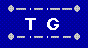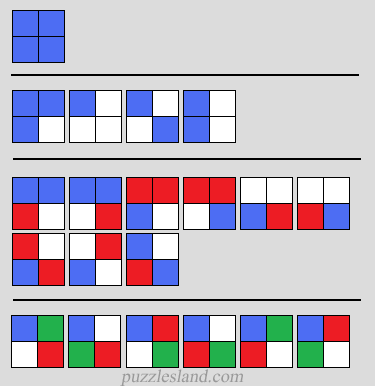## Squarematch - counting tilesFirst let's look at using various numbers of colors:

1 color - 1 way (all corners same color)

2 colors - 4 ways
(2 with 3 and 1, 1 checkerboard, and 1 stripes)

3 colors - 9 ways (6 with a stripe, 3 checkerboard)

4 colors - 6 ways
(put color #1 top left. 3 choices for bottom right, and 2 ways to put the others)

Then for each of those there are various ways to choose the colors.
If there are N colors available, we can choose:

1 color in Nc1 = N ways
2 colors in Nc2 = N (N-1) / 2 ways
3 colors in Nc3 = N (N-1) (N-2) / 6 ways
4 colors in Nc4 = N (N-1) (N-2) (N-3) / 24 ways

So for various numbers of colors we can color the 4 sections of a square in ...

1 color: (1c1 * 1) = 1 tile
2 colors: (2c1 * 1) + (2c2 * 4) = 6 tiles
3 colors: (3c1 * 1) + (3c2 * 4) + (3c3 * 9) = 24 tiles
4 colors: (4c1 * 1) + (4c2 * 4) + (4c3 * 9) + (4c4 * 6) = 70 tiles
5 colors: (5c1 * 1) + (5c2 * 4) + (5c3 * 9) + (5c4 * 6) = 165 tiles

This type of counting is addressed by Burnside's Lemma.

For a general formula for n colors see:
OEIS Sequence A006528 (n^4 + n^2 + 2*n)/4
and for a variation where reflections as well as rotations are not counted:
OEIS Sequence A002817 n*(n+1)*(n^2+n+2)/8